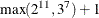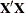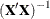### FOCUS AREAS

Contents | SAS Program | PDF

# The %MktDes Macro

## Introduction

The %MktDes autocall macro creates efficient experimental designs. You usually do not need to call the %MktDes macro directly. Instead, you usually use the %MktEx autocall macro, which calls the %MktDes macro as one of its many tools. At the heart of the %MktDes macro are the PLAN, FACTEX, and OPTEX procedures. PROC PLAN creates full-factorial designs. PROC FACTEX creates fractional-factorial designs. You can use both procedures to create a candidate set for PROC OPTEX to search. The %MktDes macro is used instead of calling those procedures directly because the %MktDes macro has a simpler syntax. To use the macro, you specify the names of the factors, the number of levels for each factor, and the number of runs that you want in your final design. For example, you can create a design in 18 runs with two 2-level factors (`X1` and `X2`) and three 3-level factors (`X3`, `X4`, and `X5`) as follows:

```%mktdes(factors=x1-x2=2 x3-x5=3, n=18)
```

You can optionally specify interactions that you want to estimate. The macro creates a candidate design in which every effect that you want to estimate is estimable but the candidate design is bigger than you want. By default, the candidate set is saved in a SAS data set called `Cand1`. The macro then uses PROC OPTEX to search the candidate design for an efficient final design. By default, the final experimental design is saved in a SAS data set called `Design`.

When the full-factorial design is small (by default fewer than 2,189 runs, although sizes up to 5,000 or 6,000 runs are reasonably small), the experimental design problem is straightforward. First, the %MktDes macro uses PROC PLAN to create a full-factorial candidate set. Next, PROC OPTEX searches the full-factorial candidate set. For very small problems (a few hundred candidates), PROC OPTEX often finds the optimal design. For larger problems, although PROC OPTEX might not find the absolute best design, with sufficient iterations (for example, MAXITER=100 or more) it finds very good designs. Run times usually range from a few seconds to a few minutes, but they can be longer.

### %MktDes Macro Syntax

%MktDes(FACTORS=factor-list <, optional arguments>)

### Required Argument

FACTORS=factor-list

specifies the factors and the number of levels for each factor. The following simple example creates a design that has ten 2-level factors:

```%mktdes(factors=x1-x10=2)
```

First, a factor list, which is a valid SAS variable list, is specified. The factor list must be followed by an equal sign and an integer, which gives the number of levels. Multiple lists can be specified. For example, to create five 2-level factors, five 3-level factors, and five 5-level factors, specify

```%mktdes(factors=x1-x5=2 x6-x10=3 x11-x15=5)
```

By default, this macro creates each factor in a fractional-factorial candidate set from a minimum number of pseudo-factors. Pseudo-factors are not output; they are used to create the factors of interest and then discarded. For example, when NLEV=2, a three-level factor `X1` is created from two 2-level pseudo-factors (`_1` and `_2`) and their interaction by coding down:

```(_1=1, _2=1) -> x1=1
(_1=1, _2=2) -> x1=2
(_1=2, _2=1) -> x1=3
(_1=2, _2=2) -> x1=1
```

This creates imbalance—the 1 level appears twice as often as the 2 and 3 levels. You can obtain somewhat better balance by instead using three pseudo-factors. You can specify the number of pseudo-factors in parentheses after the number of levels, for example, as follows:

```%mktdes(factors=x1-x5=2 x6-x10=3(3))
```

The levels 1 to 8 are coded down to 1 2 3 1 2 3 1 3, which is better balanced. The cost is that candidate-set size might increase and efficiency might actually decrease. Some researchers are willing to sacrifice a little bit of efficiency in order to achieve better balance.

### Optional Arguments

BIG=n

specifies the size at which the candidate set is considered to be big. By default, BIG=2188. If the size of the full-factorial design is less than or equal to this size, and if PROC PLAN is in the RUN= argument list, the macro uses PROC PLAN instead of PROC FACTEX to create the candidate set. The default of 2,188 is. Specifying values as large as BIG=6000 or even slightly larger is often reasonable. However, run time is slower as the size of the candidate set increases. The %MktEx macro coordinate-exchange algorithm usually works better than a candidate-set search when the full-factorial design has more than several thousand runs.

CAND=SAS-data-set

specifies the output data set that contains the candidate design (from PROC FACTEX or PROC PLAN). The default name is `Cand`, followed by the step number—for example, `Cand1` for step 1, `Cand2` for step 2, and so on. You should use this option only when you are reading an external candidate set. When you specify STEP= argument values greater than 1, the macro assumes that the default candidate set names, `Cand1`, `Cand2`, and so on, were used in previous steps. Specify only a data set name and no data set options.

CLASSOPTS=options

specifies CLASS statement options in PROC OPTEX. By default, CLASSOPTS=PARAM=ORTHREF. You should probably never change this option.

CODING=name

specifies the CODING= option in PROC OPTEX.

EXAMINE=<I> <V>

specifies the matrices that you want to examine. EXAMINE=I displays the information matrix,; EXAMINE=V displays the variance matrix,; and EXAMINE=I V displays both. These matrices are not displayed by default.

FACOPTS=options

specifies statement options in PROC FACTEX.

GENERATE=options

specifies the GENERATE statement options in PROC OPTEX. By default, additional options are not added to the GENERATE statement.

INTERACT=interaction-list

specifies interactions that must be estimable. By default, no interactions are guaranteed to be estimable. Examples include the following:

```interact=x1*x2
interact=x1*x2 x3*x4*x5
interact=x1|x2|x3|x4|x5@2
interact=@2}
```

The interaction syntax is similar to that of PROC GLM and many other SAS/STAT procedures. It uses "*" for simple interactions (`x1*x2` is the interaction between `x1` and `x2`), "|" for main effects and interactions (`x1|x2|x3` is the same as `x1 x2 x1*x2 x3 x1*x3 x2*x3 x1*x2*x3`), and "@" to eliminate higher-order interactions (`x1|x2|x3@2` eliminates `x1*x2*x3` and is the same as `x1 x2 x1*x2 x3 x1*x3 x2*x3`). The specification "`@2`" creates main effects and two-way interactions. Only "`@`" values of `2` or `3` are permitted. If you specify "@2" by itself, a resolution V design is requested when PROC FACTEX is run.

ITER=n
MAXITER=n

specifies the ITER= option in PROC OPTEX, which creates n designs. By default, ITER=10.

KEEP=n

specifies the KEEP= option in PROC OPTEX, which keeps the n best designs. By default, KEEP=5.

NLEV=n

specifies the number of levels from which factors are constructed through pseudo-factors and coding down. The value must be a prime or a power of a prime: 2, 3, 4, 5, 7, 8, 9, 11, and so on. This argument is used with PROC FACTEX as follows:

```factors factors / nlev=&nlev;
```

By default, the macro uses the minimum prime or power of a prime from the FACTORS= argument list, or 2 if no suitable value is found.

METHOD=name

specifies the METHOD= search method option in PROC OPTEX. By default, METHOD=M_FEDOROV (modified Fedorov).

N=n | SATURATED

specifies the N= option in PROC OPTEX, which is the number of runs in the final design. The default is the PROC OPTEX default and depends on the problem. You usually do not want to use the default. Instead, you should pick a value by using as guidance the information produced by the %MktRuns macro. N=SATURATED creates a design that has the minimum number of runs.

OPTIONS=options-list

specifies binary options. You can specify one or more of the following values:

CHECK

checks the efficiency of a given design, specified in the CAND= argument.

NOCODE

suppresses the display of the PROC PLAN, PROC FACTEX, and PROC OPTEX code.

ALLCODE

displays all code, including code that will not be run.

By default, none of these binary options are specified.

OTHERFAC=variable-list

specifies other terms to mention in the FACTORS statement in PROC FACTEX. These terms are not guaranteed to be estimable. By default, there are no other factors.

OTHERINT=terms

specifies interaction terms that will be specified using PROC OPTEX only for multistep macro invocations. By default, no interactions are guaranteed to be estimable. Normally, interactions that are specified via the INTERACT= option affect the MODEL statements in both PROC FACTEX and PROC OPTEX. In multistep problems, part of an interaction might not be in a particular PROC FACTEX step. In that case, the interaction term must appear only in the PROC OPTEX step. For example, if `X1` is created in one step and `X4` is created in another, and if the `X1*X4` interaction must be estimable, specify OTHERINT=X1*X4 in the final step, which runs PROC OPTEX. The following steps create the design:

```%mktdes(step=1, factors=x1-x3=2, n=30, run=factex)

%mktdes(step=2, factors=x4-x6=3, n=30, run=factex)

%mktdes(step=3, factors=x7-x9=5, n=30, run=factex optex,
otherint=x1*x4)
```
OUT=SAS-data-set

specifies the output experimental design (from PROC OPTEX). By default, OUT=`Design`.

PROCOPTS=options

specifies PROC OPTEX statement options. By default, no options are added to the PROC OPTEX statement.

RUN=procedure-list

specifies the list of procedures that the macro can run. Usually, the macro runs either PROC FACTEX or PROC PLAN and then PROC OPTEX. By default, RUN=PLAN FACTEX OPTEX. You can skip steps by omitting procedure names from this list. When both PROC PLAN and PROC FACTEX are in the list, the macro chooses between them based on the size of the full-factorial design and the value of the BIG= argument. When PROC PLAN is not in the list, the macro generates code for PROC FACTEX.

SEED=n

specifies the random number seed. By default, SEED=0, and clock time is used to make the random number seed.

SIZE=n | MIN

specifies the size of the candidate set. This argument is used together with PROC FACTEX as follows:

```size design=&size;
```
MIN

specifies the minimum size. It is recommended that you start with this value (the default) and see how large the resulting design is. If you want a larger design, specify SIZE=n.

n

increases the size of the candidate set by a factor of n. It is recommended that you base n on the value of the NLEV= argument. For example, if NLEV=2, specify SIZE=2 at each subsequent macro call. If SIZE=MIN results in a candidate set of size 128 and you specify SIZE=2 at each subsequent macro call, then those calls try to set sizes of 256, 512, 1024, and 2048. You can specify integer expressions (for example, SIZE=128*4).

By default, SIZE=MIN.

STEP=n

specifies the step number. By default, there is only one step. However, sometimes, a better design can be found by using a multistep approach. Do not specify the CAND= argument in any step of a multistep run. Consider the problem of making a design that has three 2-level factors, three 3-level factors, and three 5-level factors. The simplest approach is to do something like creating a design from 2-level factors by using pseudo-factors and coding down:

```%mktdes(factors=x1-x3=2 x4-x6=3 x7-x9=5, n=30)
```

However, for small problems like this, the following three-step approach is usually better:

```%mktdes(step=1, factors=x1-x3=2, n=30, run=factex)
%mktdes(step=2, factors=x4-x6=3, n=30, run=factex)
%mktdes(step=3, factors=x7-x9=5, n=30, run=factex optex)
```

Note, however, that the following %MktEx macro step is usually better still:

```%mktex(2 2 2 3 3 3 5 5 5, n=30)
```

The first %MktDes macro step uses PROC FACTEX to create a fractional-factorial design for the 2-level factors. The second step uses PROC FACTEX to create a fractional-factorial design for the 3-level factors and cross it with the 2-level factors. The third step uses PROC FACTEX to create a fractional-factorial design for the 5-level factors and cross it with the design for the 2- and 3-level factors and then run PROC OPTEX.

Each step globally stores two macro variables (`&class1` and `&inter1` for the first step, `&class2` and `&inter2` for the second step, and so on) that are used to construct the CLASS and MODEL statements in PROC OPTEX. When the value of the STEP= argument is greater than 1, variables from the previous steps are used in the CLASS and MODEL statements. In this example, the following PROC OPTEX code is created by the third step:

```proc optex data=Cand3;
class x1-x3 x4-x6 x7-x9 / param=orthref;
model x1-x3 x4-x6 x7-x9;
generate n=30 iter=10 keep=5 method=m_fedorov;
output out=Design;
run; quit;
```

This step uses the previously stored macro variables `&class1=x1-x3` and `&class2=x4-x6`.

WHERE=where-clause

specifies a SAS WHERE clause for the candidate design, which is used to restrict the candidates. By default, the candidate design is not restricted.

## Help Option

You can specify either of the following to display the option names and simple examples of the macro syntax:

```%mktdes(help)
%mktdes(?)
```

## %MktDes Macro Notes

This macro specifies `options nonotes` throughout most of its execution. If you want to see all the notes, submit the following statement before running the macro:

```%let mktopts = notes;
```

To see the macro version, submit the following statement before running the macro:

```%let mktopts = version;
```

## Example

The following example shows to use the %MktDes macro to find an optimal nonorthogonal design when the full-factorial design is small (108 runs):

```%mktdes(factors=x1-x2=2 x3-x5=3, n=18, maxiter=500)
```

Figure 1 shows the %MktDes macro’s output.

Figure 1: %MktDes Output

The OPTEX Procedure

Class Level Information
Class Levels Values
x1 2 1 2
x2 2 1 2
x3 3 1 2 3
x4 3 1 2 3
x5 3 1 2 3

Design Number D-Efficiency A-Efficiency G-Efficiency Average Prediction
Standard Error
1 99.8621 99.7230 98.6394 0.7081
2 99.8621 99.7230 98.6394 0.7081
3 99.8621 99.7230 98.6394 0.7081
4 99.8621 99.7230 98.6394 0.7081
5 99.8621 99.7230 98.6394 0.7081

The data set `Design` contains the design that the macro finds:

```proc print data=Design noobs;
run;
```

Figure 2 shows the results.

Figure 2: Optimal Nonorthogonal Design

x1 x2 x3 x4 x5
2 2 3 3 2
2 2 2 2 1
2 2 1 3 1
2 2 1 1 3
2 1 3 2 3
2 1 3 1 1
2 1 2 3 3
2 1 2 1 2
2 1 1 2 2
1 2 3 3 2
1 2 3 2 3
1 2 2 2 1
1 2 2 1 2
1 2 1 1 3
1 1 3 1 1
1 1 2 3 3
1 1 1 3 1
1 1 1 2 2

When the full-factorial design is larger, the macro uses PROC FACTEX to create a fractional-factorial candidate set. In those cases, the methods that the %MktEx macro finds usually make better designs than the methods that the %MktDes macro finds.